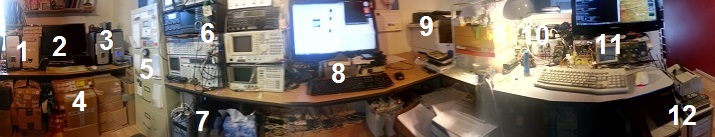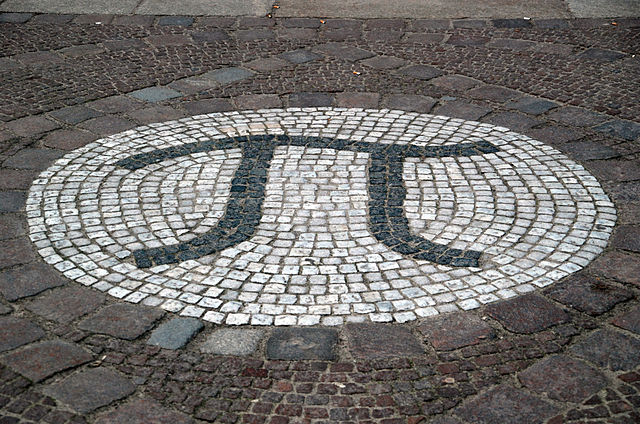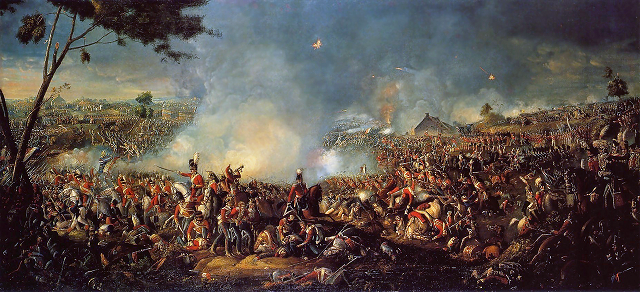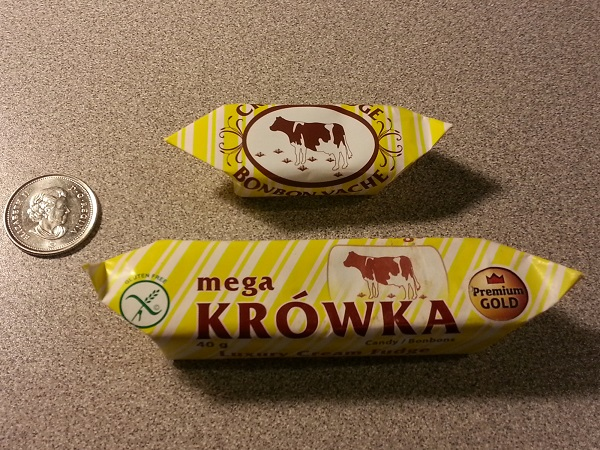Someone on Quora asked if hackers really need multiple computers. Well… I am not technically a hacker (in the bad sense of the word) as I do not use my skills for illicit purposes, but I certainly have multiple computers, as this panoramic picture taken from my home office chair demonstrates:Here is what’s in this picture:

1. Two older, dual-core workstations that I still keep hooked up for test purposes.
2. A monitor (currently off) with a KVM connecting the four computers on this desk. Under the monitor, three laptops (my current travel laptop, a still more or less current netbook, and an older laptop that I don’t really use anymore.)
3. Two more computers: my main server and its standby backup. On top, a wireless access point; behind (not visible) two network routers and several concentrators, as well as an older monochrome laser printer. Behind on the floor, there is also a UPS.
4. Underneath it all: several cardboard boxes containing vintage calculators and various bits of computer parts.
5. A filing cabinet. (On top, not seen, some radio frequency equipment, a multi-standard VHS VCR that I still occasionally use to digitize old videos, and a turntable record player.)
6. Several pieces of radio frequency test equipment, owned by one of my clients. On top (not visible) my tablet.
7. Underneath, my main workstation, with 2×24 TB (mirrored) external storage. A UPS is behind the workstation.
8. My main monitor and keyboard. Under the monitor, a photo printer, and my old smartphone (still functional, with a data-only SIM card that I keep as a backup Internet connection. My current smartphone is the one I used to take this picture.)
9. A laser printer and scanner. Underneath, under the desk, some boxes of paper, manuals, etc.
10. My “hardware” desk, with boxes of parts, a soldering iron, a test power supply, a couple of multimeters and other equipment. Under the desk (not seen) more computer parts and more radio equipment.
11. My secondary monitor and keyboard. An oscilloscope is sitting under the monitor.
12. Two more computers: an older Windows 98 machine that I keep around as it can connect to legacy hardware (including the old “winprinter” style laser printer seen here, as well as an EPROM programmer) and a backup of my main workstation. A UPS is also visible.

Not seen in this picture (behind me and/or above) are bookshelves full of technical books and literature, folders containing MSDN subscription CDs/DVDs, three additional older computers (not hooked up, but functional) and additional computer parts, lots of cables, etc.

Most of this equipment is “in use”. Out of the 7 desktop computers shown, three are currently powered (but two are powered 24/7, a server and my main workstation.)

There is a particularly neat way to derive Schrödinger’s equation, and to justify the “canonical substitution” rules for replacing energy and momentum with corresponding operators when we “quantize” an equation.

Take a particle in a potential. Its energy is given by

$$E=\frac{{\bf p}^2}{2m}+V({\bf x}),$$

or

$$E-\frac{{\bf p}^2}{2m}-V({\bf x})=0.$$

Now multiply both sides this equation by the formula $$e^{i({\bf p}\cdot{\bf x}-Et)/\hbar}$$. We note that this exponential expression cannot ever be zero if the part in the exponent that’s in parentheses is real:

$$\left[E-\frac{{\bf p}^2}{2m}-V({\bf x})\right]e^{i({\bf p}\cdot{\bf x}-Et)/\hbar}=0.$$

So far so good. But now note that

$$Ee^{i({\bf p}\cdot{\bf x}-Et)/\hbar}=i\hbar\frac{\partial}{\partial t}e^{i({\bf p}\cdot{\bf x}-Et)/\hbar},$$

and similarly,

$${\bf p}^2e^{i({\bf p}\cdot{\bf x}-Et)/\hbar}=-\hbar^2{\boldsymbol\nabla}e^{i({\bf p}\cdot{\bf x}-Et)/\hbar}.$$

This allows us to rewrite the previous equation as

$$\left[i\hbar\frac{\partial}{\partial t}+\hbar^2\frac{{\boldsymbol\nabla}^2}{2m}-V({\bf x})\right]e^{i({\bf p}\cdot{\bf x}-Et)/\hbar}=0.$$

Or, writing $$\Psi=e^{i({\bf p}\cdot{\bf x}-Et)/\hbar}$$ and rearranging:

$$i\hbar\frac{\partial}{\partial t}\Psi=-\hbar^2\frac{{\boldsymbol\nabla}^2}{2m}\Psi+V({\bf x})\Psi,$$

which is the good old Schrödinger equation.

The method works for an arbitrary, generic Hamiltonian, too. Given

$$H({\bf p})=E,$$

we can write

$$\left[E-H({\bf p})\right]e^{i({\bf p}\cdot{\bf x}-Et)/\hbar}=0,$$

which is equivalent to

$$\left[i\hbar\frac{\partial}{\partial t}-H(-i\hbar{\boldsymbol\nabla})\right]\Psi=0.$$

So if this equation is identically satisfied for a classical system with Hamiltonian $$H$$, what’s the big deal about quantum mechanics? Well… a classical system satisfies $$E-H({\bf p})=0$$, where $$E$$ and $${\bf p}$$ are eigenvalues of the differential operators $$i\hbar\partial/\partial t$$ and $$-i\hbar{\boldsymbol\nabla}$$, respectively. Schrödinger’s equation, on the other hand, remains valid in the general case, not just for the eigenvalues.

Having just finished work on a major project milestone, I took it easy for a few days, allowing myself to spend time thinking about other things. That’s when I encountered an absolutely neat problem on Quora.Someone asked a seemingly innocuous number theory question: are there two positive integers such that one is exactly the π-th power of the other?

Now wait a minute, you ask… We know that π is a transcendental number. How can an integer raised to a transcendental power be another integer?

But then you think about $$\alpha=\log_2 3$$ and realize that although $$\alpha$$ is a transcendental number, $$2^\alpha=3$$. So why can’t we have $$n^\pi=m$$, then?

As it turns out, we (probably) cannot, but the reason is subtle and it relies on a very important, but unproven conjecture from transcendental number theory.

But first, let us rewrite the equation by taking its logarithm:

$$\pi\log n = \log m.$$

We can also divide both sides by $$\log n$$, which leads to

$$\pi = \frac{\log m}{\log n}=\log_n m,$$

but it turns out to be not very helpful. However, squaring the equation will help, as we shall shortly see:

$$\pi^2\log^2 n=\log^2 m.$$

Can this equation ever by true for positive integers $$n$$ and $$m$$, other than the trivial solution $$n=m=1$$, that is?

To see why it cannot be the case, let us consider the following triplet of numbers:

$$(i\pi,\log n,\log m),$$

and their exponents,

$$(e^{i\pi}=-1, e^{\log n}=n, e^{\log m}=m).$$

The three numbers $$(i\pi,\log n,\log m)$$ are linearly independent over $${\mathbb Q}$$ (that is, the rational numbers). What this means is that there are no rational numbers $$A, B, C, D$$ such that $$Ai\pi+B\log n+C\log m + D=0$$. This is easy to see as the ratio of $$\log n$$ and $$\log m$$ is supposed to be transcendental but both numbers are real, whereas $$i\pi$$ is imaginary.

On the other hand, their exponents are all rational numbers ($$-1, n, m$$). And this is where the unproven conjecture, Schanuel’s conjecture, comes into the picture. Schanuel’s conjecture says that given $$n$$ complex numbers $$(\alpha_1,\alpha_2,…,\alpha_n)$$ that are linearly independent over the rationals, out of the $$2n$$ numbers $$(\alpha_1,…,\alpha_n,e^{\alpha_1},…,e^{\alpha_n})$$, at least $$n$$ will be transcendental numbers that are algebraically independent over $${\mathbb Q}$$. That is, there is no algebraic expression involving roots and powers of the $$\alpha_i$$, $$e^{\alpha_i}$$, and rational numbers that will yield 0.

The equation $$\pi^2\log^2 n=\log^2 m$$, which we can rewrite as

$$(i\pi)^2\log^2 n + \log^2 m=0,$$

is just such an equation, and it can never be true.

I wish I could say that I came up with this solution but I didn’t. I was this close: I was trying to apply Schanuel’s conjecture, and I was of course using the fact that $$\pi=-i\log -1$$. But I did not fully appreciate the implications and meaning of Schanuel’s conjecture, so I was applying it improperly. Fortunately, another Quora user saved the day.

Still I haven’t had this much fun with pure math (and I haven’t learned this much pure math all at once) in years.

Today is the 200th anniversary of the Battle of Waterloo, the final, decisive battle that ended Napoleon’s second, 100-day reign.People occasionally call the Napoleonic Wars World War 0: like the two World Wars of the 20th century, this war, too, was global in scope (especially if I include the War of 1812) and millions died.

Others suggest that there were much larger conflicts in Europe’s past, such as the Seven Years’ War. Perhaps true, but I’d argue that like the two World Wars of the 20th century, the Napoleonic Wars also had a more lasting legacy: they changed the existing world order. Before the Napoleonic Wars, Europe was still a collection of medieval, mostly multi ethnic feudal empires. The Napoleonic Wars sowed the seeds of ethnic nationalism on the one hand and constitutional democracies on the other. They gave rise, for better or for worse, to the modern ethnic nation states that characterize Europe today. Meanwhile in North America, the War of 1812 established the path that led to Canada’s nationhood.

So to me, it’s World War 0. And it ended exactly 200 years ago today. Unlike World War I, it wasn’t “the war to end all wars”, although it did bring in an unprecedented era of relative peace, prosperity and enlightenment in Europe. As a result, the 19th century became the true century of science and technology, which saw the emergence of modern cities with electric lighting, streetcars, telephone and telegraph networks, proper sanitation, functioning social institutions, modern police forces, newspapers and public education. It is too bad that in the century that followed, the century that was greeted with such hope and expectations that it was once dubbed “the century of reason”, saw ethnic nationalism take center stage and lead to unspeakable horrors.

A great many years ago, my uncle Jóska once played a joke on the family. He found a large utility scrub brush somewhere, and at the most unexpected moments, he held it under your nose and said, “Come, give a kiss to grandpa!”What he really meant, of course, is that the scrub brush looked like the grandfather of all toothbrushes. Even so, “give a kiss to grandpa” became kind of an inside joke in our family whenever we encountered something that was unusually oversized.

Like an oversized piece of caramel candy.

When I was a child, one of our favorite candies was the “cow caramel” candy, a Polish product, a caramel candy that, unlike most caramel candies, was neither sticky nor chewy; rather, it just crumbled in your mouth without sticking to your teeth or palate. Oh, and it was very tasty, too.

This “cow candy” is still manufactured, and it is routinely available even in Canada at European deli shops. Although we try to keep our candy consumption at a minimum for all the obvious reasons, my wife and I remain regular buyers.

So imagine my surprise when the other day, my wife comes home with the grandfather of cow candies: a giant, mega-size (it says so on the label) cow candy that looks just like the regular cow candy, except that it’s much bigger.

But, I can attest, just as tasty. We cut this one in half and enjoyed it earlier tonight, but not before I snapped a picture, showing the “regular” cow candy and a Canadian quarter for size comparison.